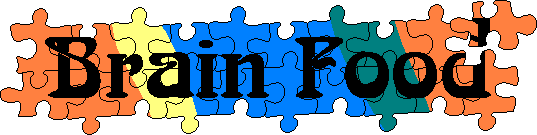Main      Site Guide### Associated Words

In each of these puzzles, a list of words is given. To solve the puzzle, think of a single word that goes with each to form a compound word (or word pair that functions as a compound word). For example, if the given words are volley, field, and bearing, then the answer would be ball, because the word ball can be added to each of the other words to form volleyball, ballfield, and ball bearing.

• back
• short
• watch

Solution

• blue
• cake
• cottage

Solution

• stool
• powder
• ball

Solution

• big
• soil
• table

Solution

• cuff
• left

Solution

• motion
• poke
• down

Solution

• light
• hot
• check

Solution

• light
• hot
• back

Solution

• wood
• liquor
• luck

Solution

• drop
• off
• stand

Solution